Excel MAX Function & MIN Function | CustomGuide# Excel MAX Function & MIN Function

How to Use MIN and MAX Functions in Microsoft Excel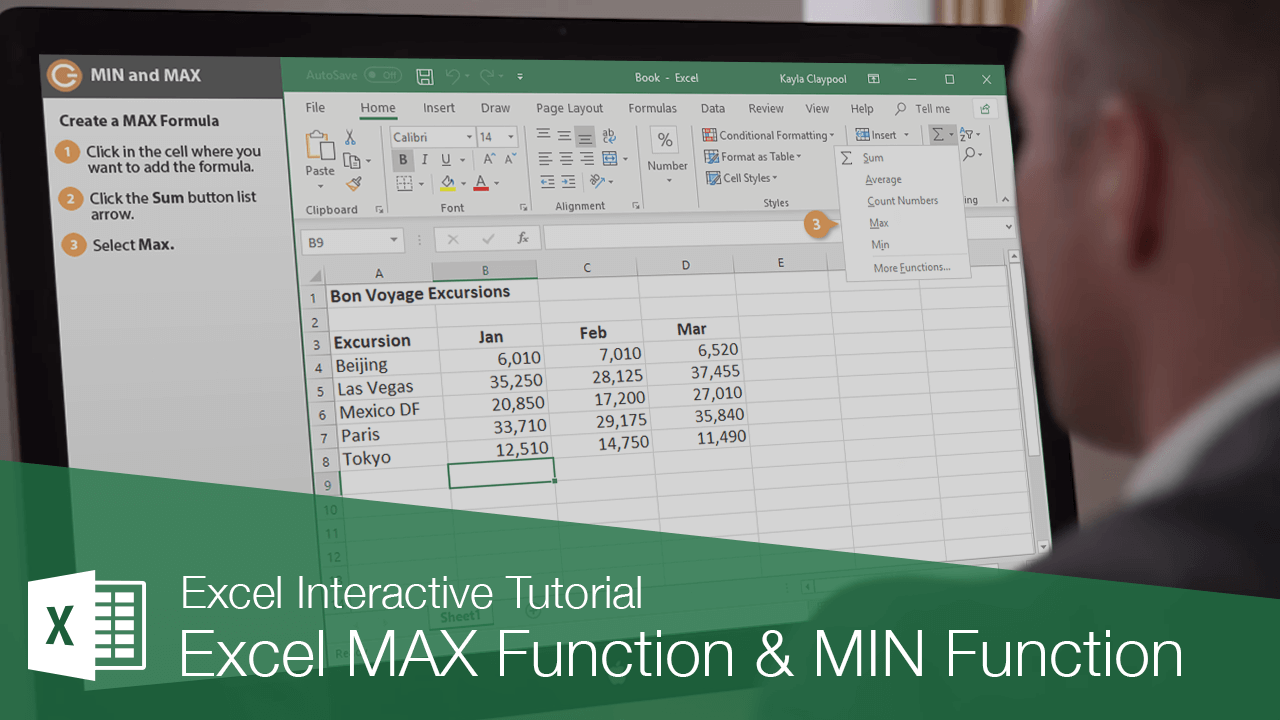The Excel MAX function can tell you what the largest numeric value is in a range of cells. The opposite of MAX is the MIN function, which returns the smallest numeric value in a range of cells.

### Create a MAX Formula

1. Click in the cell where you want to add the formula.
2. Click the Sum button list arrow.
3. Select Max.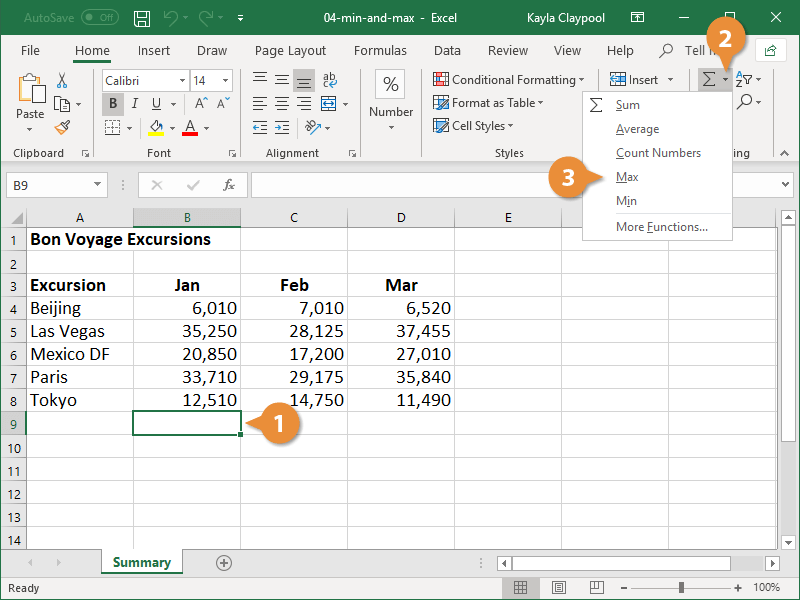4. If necessary, select the desired cell range.
5. Click Enter or press the Enter key.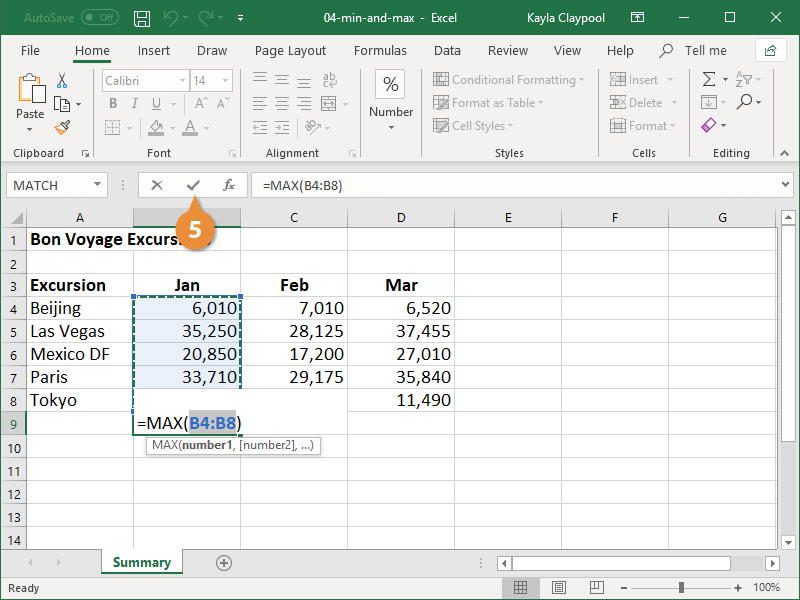Excel adds the MAX formula and displays the biggest number from the specified range.

### Create a MIN Formula

1. Click in the cell where you want to add the formula.
2. Click the AutoSum button list arrow.
3. Select Min.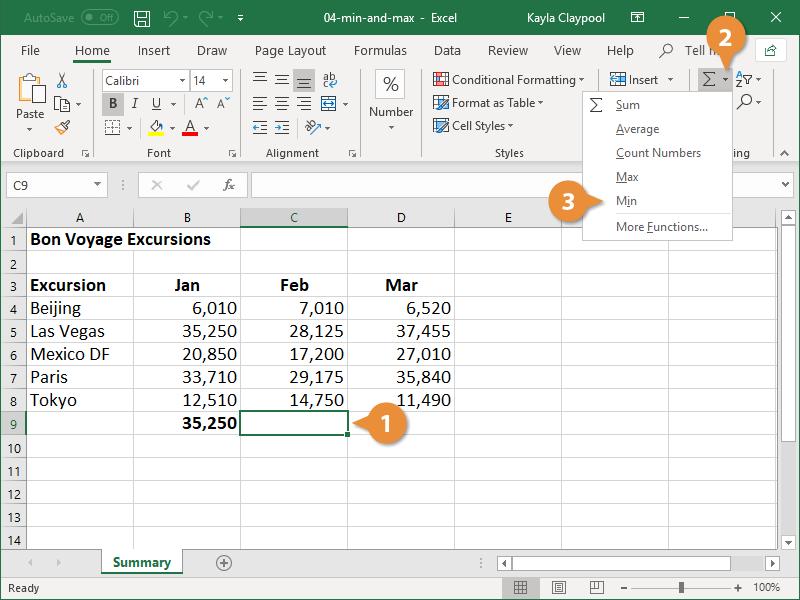4. If necessary, select the desired cell range.
5. Click Enter or press the Enter key.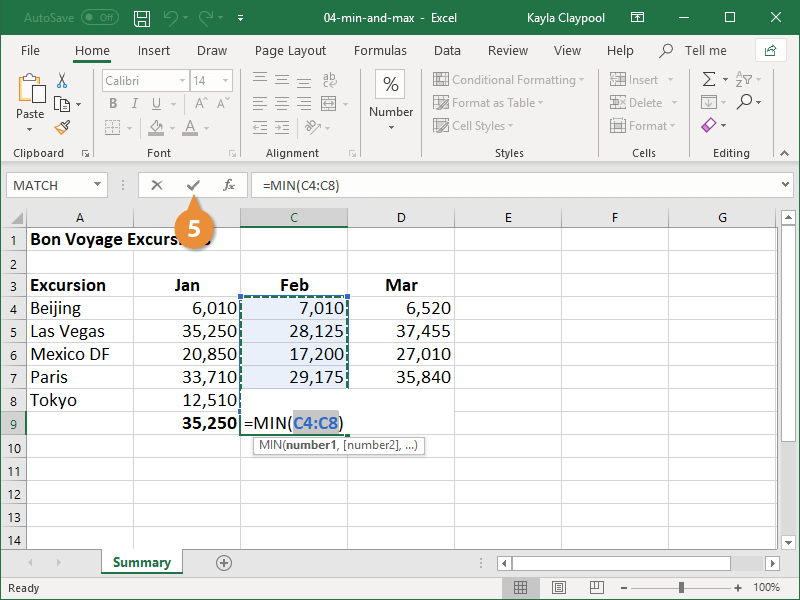Excel adds the MIN formula and displays the smallest number from the specified range.